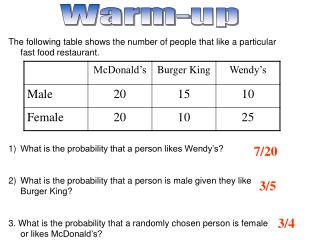# Warm-up - PowerPoint PPT PresentationDownload PresentationWarm-up

Warm-upDownload Presentation## Warm-up

- - - - - - - - - - - - - - - - - - - - - - - - - - - E N D - - - - - - - - - - - - - - - - - - - - - - - - - - -
##### Presentation Transcript

1. Warm-up • The following table shows the number of people that like a particular fast food restaurant. • What is the probability that a person likes Wendy’s? • What is the probability that a person is male given they like Burger King? • 3. What is the probability that a randomly chosen person is female or likes McDonald’s? 7/20 3/5 3/4

2. Probability Independent vs. Dependent events

3. Independent Events • Two events A and B, are independent if the fact that A occurs does not affect the probability of B occurring. • Examples- Landing on heads from two different coins, rolling a 4 on a die, then rolling a 3 on a second roll of the die. • Probability of A and B occurring: P(A and B) = P(A)  P(B)

4. Experiment 1 • A coin is tossed and a 6-sided die is rolled. Find the probability of landing on the head side of the coin and rolling a 3 on the die. • P (head)=1/2 • P(3)=1/6 • P (head and 3)=P (head)  P(3) =1/2  1/6 = 1/12

5. Experiment 2 • A card is chosen at random from a deck of 52 cards. It is then replaced and a second card is chosen. What is the probability of choosing a jack and an eight? • P (jack)= 4/52 • P (8)= 4/52 • P (jack and 8)= 4/52  4/52 = 1/169

6. Experiment 3 • A jar contains three red, five green, two blue and six yellow marbles. A marble is chosen at random from the jar. After replacing it, a second marble is chosen. What is the probability of choosing a green and a yellow marble? • P (green) = 5/16 • P (yellow) = 6/16 • P (green and yellow) = P (green)  P (yellow) = 15 / 128

7. Experiment 4 • A school survey found that 9 out of 10 students like pizza. If three students are chosen at random with replacement, what is the probability that all three students like pizza? • P (student 1 likes pizza) = 9/10 • P (student 2 likes pizza) = 9/10 • P (student 3 likes pizza) = 9/10 • P (student 1 and student 2 and student 3 like pizza) = 9/10  9/10  9/10 = 729/1000

8. Dependent Events • Two events A and B, are dependent if the fact that A occurs affects the probability of B occurring. • Examples- Picking a blue marble and then picking another blue marble if I don’t replace the first one. • Probability of A and B occurring: P(A and B) = P(A)  P(B|A)

9. Experiment 1 • A jar contains three red, five green, two blue and six yellow marbles. A marble is chosen at random from the jar. A second marble is chosen without replacing the first one. What is the probability of choosing a green and a yellow marble? P (green) = 5/16 P (yellow given green) = 6/15 P (green and then yellow) = P (green)  P (yellow) = 1/8

10. Experiment 2 • An aquarium contains 6 male goldfish and 4 female goldfish. You randomly select a fish from the tank, do not replace it, and then randomly select a second fish. What is the probability that both fish are male? • P (male) = 6/10 • P (male given 1st male) = 5/9 • P (male and then, male) = 1/3

11. Experiment 3 • A random sample of parts coming off a machine is done by an inspector. He found that 5 out of 100 parts are bad on average. If he were to do a new sample, what is the probability that he picks a bad part and then, picks another bad part if he doesn’t replace the first? • P (bad) = 5/100 • P (bad given 1st bad) = 4/99 • P (bad and then, bad) = 1/495

12. Independent vs. Dependent Determining if 2 events are independent

13. Independent Events • Two events are independent if the following are true: P(A|B) = P(A)  P(B|A) = P(B)  P(A AND B) = P(A) ⋅ P(B) • To show 2 events are independent, you must prove one of the above conditions.

14. Experiment 1 • Let event G  = taking a math class. Let event H  = taking a science class. Then, G AND H  = taking a math class and a science class. • Suppose P(G) = 0.6, P(H) = 0.5, and P(G AND H) = 0.3. • Are G and H independent?

15. Experiment 2 • In a particular college class, 60% of the students are female. 50% of all students in the class have long hair. 45% of the students are female and have long hair. Of the female students, 75% have long hair. • Let F be the event that the student is female. Let L be the event that the student has long hair. • One student is picked randomly. Are the events of being female and having long hair independent?

16. Approach #2 • If they are independent, P(L|F) should equal P(L). • 0.75 ≠ 0.5

17. Homework Practice Worksheet